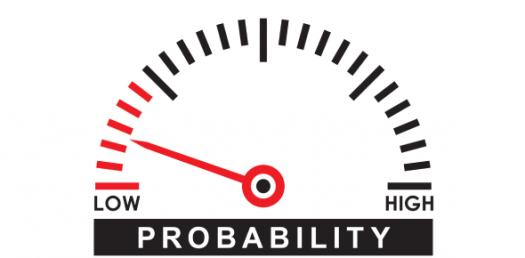# Take This Quiz To Test Your Probability Knowledge!

10 Questions | Total Attempts: 189SettingsWhat do you know about probability? The mathematics field of probability possesses it's our rules, laws, and definitions, which you may use to figure out the probability of outcomes, events, or combinations of outcomes and events. To determine the probability, add, subtract, multiply, or divide the probabilities of the original outcomes and events. Check out this quiz to see what you know.

• 1.
There were 6 purple socks and 4 orange socks in a drawer.  Jill picked one sock without looking and then another without looking (or replacing the first).  What is the probability that she picked two purple socks?
• A.

1/2

• B.

1/3

• C.

1/4

• D.

3/9

• 2.
There were 6 purple socks and 4 orange socks in a drawer.  Jill picked one sock without looking and then another without looking (or replacing the first).  What is the probability that she picked two orange socks?
• A.

2/15

• B.

4/10

• C.

7/19

• D.

3/4

• 3.
There are 10 boxes in a grab bag.  The boxes are identitcal except that 7 of the boxes contain \$20 bills.  A contest winner gets to pick two boxes from the grab bag.  What is the probability of them getting two \$20 bill boxes?
• A.

8/15

• B.

7/15

• C.

7/10

• D.

1/2

• 4.
There are 10 boxes in a grab bag.  The boxes are identitcal except that 7 of the boxes contain \$20 bills.  A contest winner gets to pick two boxes from the grab bag.  What is the probability of them getting one box with a \$20 bill and one box without?
• A.

11/30

• B.

2/15

• C.

7/30

• D.

6/15

• 5.
One letter is randomly selected from the word MATH and a second letter is randomly selected from the word PROBABILITY.  Assuming that Y is a consonant and not a vowel, what is the probability that both letters selected will be VOWELS?
• A.

1/10

• B.

1/11

• C.

1/12

• D.

2/3

• 6.
One letter is randomly selected from the word MATH and a second letter is randomly selected from the word PROBABILITY.  Assuming that Y is a consonant and not a vowel, what is the probability that both letters selected will be CONSONANTS?
• A.

2/11

• B.

3/22

• C.

16/33

• D.

21/44

• 7.
A test has two multiple choice questions, each with 5 answer choices.  What is the probability of guessing the correct answer to BOTH questions?
• A.

1/5

• B.

1/2

• C.

1/25

• D.

1/10

• 8.
A test has three multiple choice questions, each with 4 answer choices.  What is the probability of guessing the correct answer to BOTH questions?
• A.

1/4

• B.

1/64

• C.

1/12

• D.

1/32

• 9.
A test has five multiple choice questions, each with 2 answer choices.  What is the probability of guessing the correct answer to BOTH questions?
• A.

1/4

• B.

1/32

• C.

1/12

• D.

1/2

• 10.
A test has two multiple choice questions, each with 4 answer choices.  What is the probability of guessing the correct answer to one question and an incorrect answer to the other?
• A.

1/4

• B.

1/8

• C.

3/16

• D.

1/2

Related TopicsBack to top